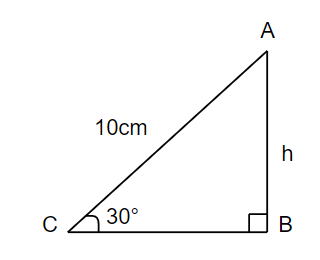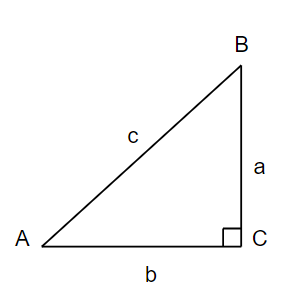QUESTION

# Find the value of $h$ in $\Delta ABC$.Hint: First of all recollect the trigonometric ratios like sin, cos, tan etc and see which is the useful one for finding $h$ of a given triangle. Preferably, use the formula for $\sin 30{}^\circ$ in a given triangle.

In the question, we have to find the value of $h$ in $\Delta ABC$. Before proceeding with the question, let us first learn about right triangles and its trigonometric ratios. A right triangle is a type of triangle that has one angle that measures $90{}^\circ$. A right triangle $ABC$ is given below, which is right angled at $C$ and has sides as, $a,b$ and $c$.We use trigonometry to find the angles and sides of the right triangle. The various functions in trigonometry are sine, cosine, tangent, cosecant, secant and cotangent. They are simply one side of a right triangle divided by another. If we take angle $A$ of the above triangle, then side $BC$ would be perpendicular, side $AC$ would be the base and side $AB$ would be the hypotenuse. So, we can write it as,
\begin{align} & \sin A=\dfrac{perpendicular}{hypotenuse}=\dfrac{a}{c} \\ & \csc A=\dfrac{hypotenuse}{perpendicular}=\dfrac{c}{a} \\ & \cos A=\dfrac{base}{hypotenuse}=\dfrac{b}{c} \\ & \sec A=\dfrac{hypotenuse}{base}=\dfrac{c}{b} \\ & \tan A=\dfrac{perpendicular}{base}=\dfrac{a}{b} \\ & \cot A=\dfrac{base}{perpendicular}=\dfrac{b}{a} \\ \end{align}
Now, let us consider our question. We can see that $\angle C=30{}^\circ ,AC=10cm$ and $AB=h$.To find the value of $h$, we have to take a trigonometric ratio such that it includes angle $30{}^\circ ,h$ and $AC=10cm$. We know that, $\sin \theta =\dfrac{perpendicular}{hypotenuse}$, so we get, $\sin C=\dfrac{AB}{AC}$.
By substituting $C=30{}^\circ ,AB=h$ and $AC=10cm$, we get, $\sin 30{}^\circ =\dfrac{h}{10}$.
We know that $\sin 30{}^\circ =\dfrac{1}{2}$, so we get,
\begin{align} & \dfrac{1}{2}=\dfrac{h}{10} \\ & \Rightarrow 10=2h \\ & \Rightarrow h=\dfrac{10}{2} \\ & \Rightarrow h=5cm \\ \end{align}
Hence, we get the value of $h$ as $5cm$ in $\Delta ABC$.
Note: In these type of questions, the students are advised to use the trigonometric ratios among $\sin \theta ,\cos \theta$ and $\tan \theta$, which gives the unknown side and the known sides of a triangle, so that we don’t have to do the extra effort of using the Pythagoras theorem. Also the students are advised to remember the values of $\sin \theta ,\cos \theta$ and $\tan \theta$ at the general angles like $\theta =0{}^\circ ,30{}^\circ ,45{}^\circ ,60{}^\circ$ and $90{}^\circ$.